MathScore EduFighter is one of the best math games on the Internet today. You can start playing for free!

## Perimeter and Area of Composite Figures - Sample Math Practice Problems

The math problems below can be generated by MathScore.com, a math practice program for schools and individual families. References to complexity and mode refer to the overall difficulty of the problems as they appear in the main program. In the main program, all problems are automatically graded and the difficulty adapts dynamically based on performance. Answers to these sample questions appear at the bottom of the page. This page does not grade your responses.

See some of our other supported math practice problems.

### Complexity=2, Mode=align-grid

Find the perimeter and area of each figure. Each unit is 1 cm. Be sure to label your answers in "cm". Type cm2 as "sq cm"

 1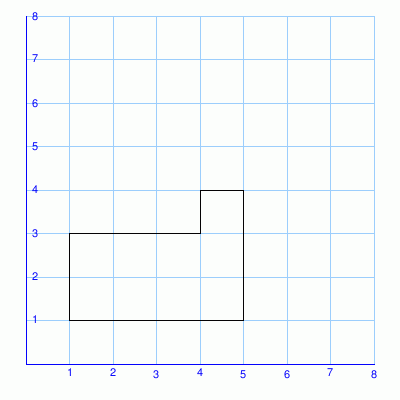perimeter = area = 2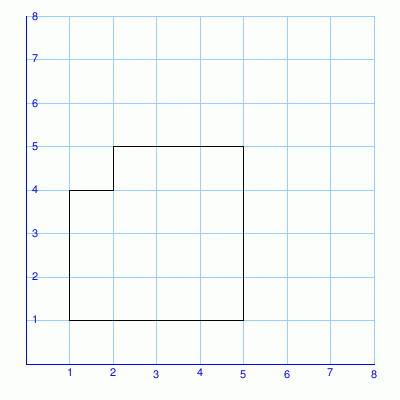perimeter = area =

### Complexity=3, Mode=align-grid

Find the perimeter and area of each figure. Each unit is 1 cm. Be sure to label your answers in "cm". Type cm2 as "sq cm"

 1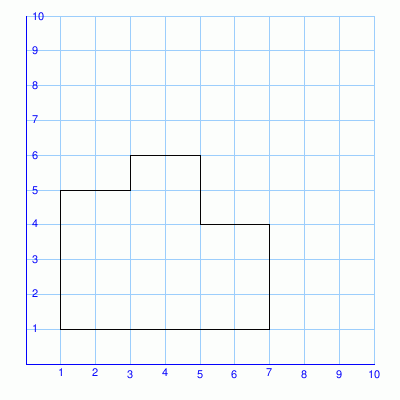perimeter = area = 2perimeter = area =

### Complexity=2, Mode=jagged-grid

Find the perimeter and area of each figure. Each unit is 1 cm. Be sure to label your answers in "cm". Type cm2 as "sq cm"

 1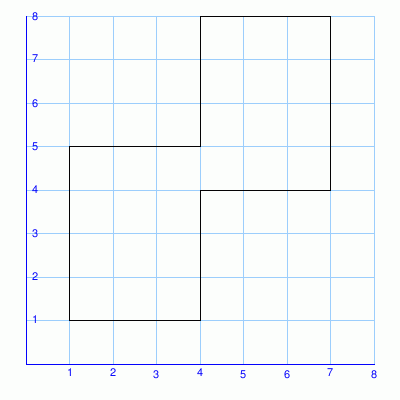perimeter = area = 2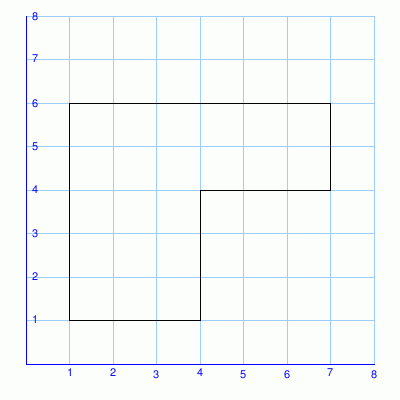perimeter = area =

### Complexity=3, Mode=jagged-grid

Find the perimeter and area of each figure. Each unit is 1 cm. Be sure to label your answers in "cm". Type cm2 as "sq cm"

 1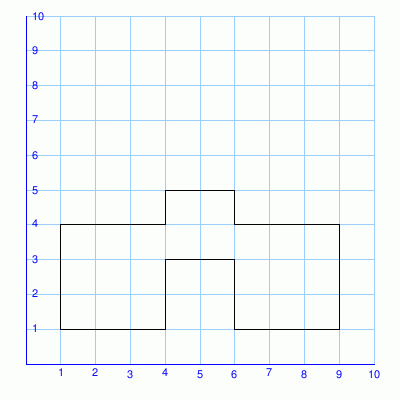perimeter = area = 2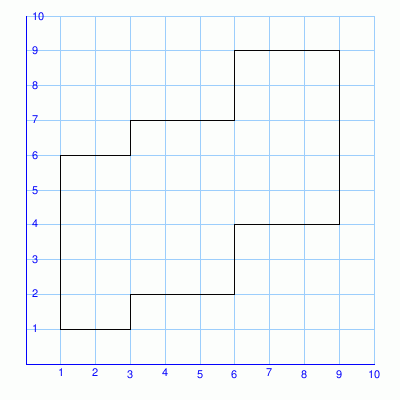perimeter = area =

### Complexity=2, Mode=align

Find the perimeter and area of each figure. Use labels.
All figures are made of rectangles.

 1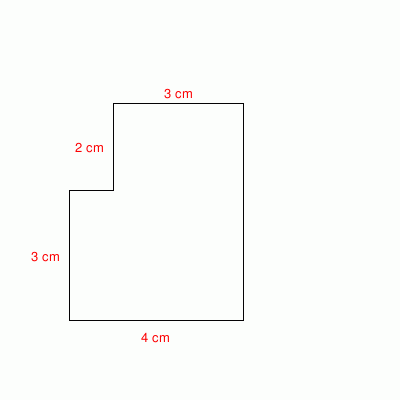perimeter = area = 2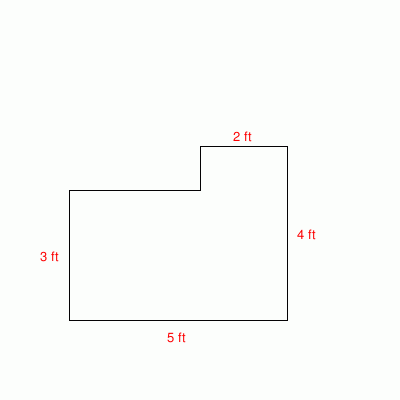perimeter = area =

### Complexity=3, Mode=align

Find the perimeter and area of each figure. Use labels.
All figures are made of rectangles.

 1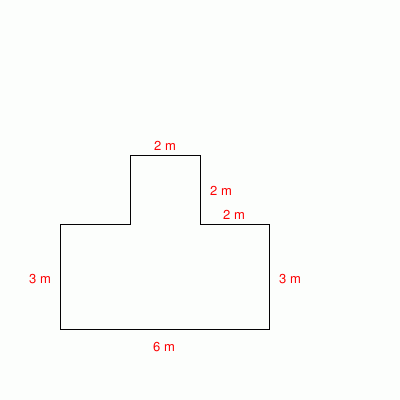perimeter = area = 2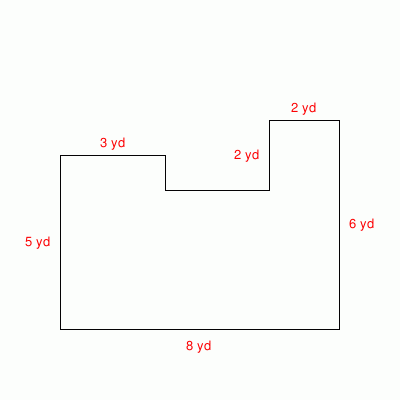perimeter = area =

### Complexity=2, Mode=align-grid

Find the perimeter and area of each figure. Each unit is 1 cm. Be sure to label your answers in "cm". Type cm2 as "sq cm"

1perimeter =
area =
Solution
To get the perimeter, add up the lengths of all the sides.
Perimeter = 3 cm + 1 cm + 1 cm + 3 cm + 4 cm + 2 cm = 14 cm

Looking at this figure, you can see that the figure is made up of rectangles.
To get the figure's area, add up the areas of the smaller rectangles.
Area = (3 cm * 2 cm) + (1 cm * 3 cm) = 9 sq cm
2perimeter =
area =
Solution
To get the perimeter, add up the lengths of all the sides.
Perimeter = 1 cm + 1 cm + 3 cm + 4 cm + 4 cm + 3 cm = 16 cm

Looking at this figure, you can see that the figure is made up of rectangles.
To get the figure's area, add up the areas of the smaller rectangles.
Area = (1 cm * 3 cm) + (3 cm * 4 cm) = 15 sq cm

### Complexity=3, Mode=align-grid

Find the perimeter and area of each figure. Each unit is 1 cm. Be sure to label your answers in "cm". Type cm2 as "sq cm"

1perimeter =
area =
Solution
To get the perimeter, add up the lengths of all the sides.
Perimeter = 2 cm + 1 cm + 2 cm + 2 cm + 2 cm + 3 cm + 6 cm + 4 cm = 22 cm

Looking at this figure, you can see that the figure is made up of rectangles.
To get the figure's area, add up the areas of the smaller rectangles.
Area = (2 cm * 4 cm) + (2 cm * 5 cm) + (2 cm * 3 cm) = 24 sq cm
2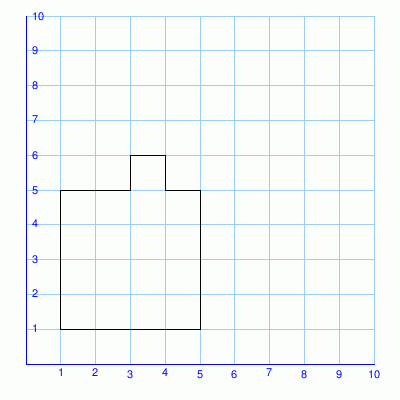perimeter =
area =
Solution
To get the perimeter, add up the lengths of all the sides.
Perimeter = 2 cm + 1 cm + 1 cm + 1 cm + 1 cm + 4 cm + 4 cm + 4 cm = 18 cm

Looking at this figure, you can see that the figure is made up of rectangles.
To get the figure's area, add up the areas of the smaller rectangles.
Area = (2 cm * 4 cm) + (1 cm * 5 cm) + (1 cm * 4 cm) = 17 sq cm

### Complexity=2, Mode=jagged-grid

Find the perimeter and area of each figure. Each unit is 1 cm. Be sure to label your answers in "cm". Type cm2 as "sq cm"

1perimeter =
area =
Solution
To get the perimeter, add up the lengths of all the sides.
Perimeter = 3 cm + 3 cm + 3 cm + 4 cm + 3 cm + 3 cm + 3 cm + 4 cm = 26 cm

Looking at this figure, you can see that the figure is made up of rectangles.
To get the figure's area, add up the areas of the smaller rectangles.
Area = (3 cm * 4 cm) + (3 cm * 4 cm) = 24 sq cm
2perimeter =
area =
Solution
To get the perimeter, add up the lengths of all the sides.
Perimeter = 6 cm + 2 cm + 3 cm + 3 cm + 3 cm + 5 cm = 22 cm

Looking at this figure, you can see that the figure is made up of rectangles.
To get the figure's area, add up the areas of the smaller rectangles.
Area = (3 cm * 5 cm) + (3 cm * 2 cm) = 21 sq cm

### Complexity=3, Mode=jagged-grid

Find the perimeter and area of each figure. Each unit is 1 cm. Be sure to label your answers in "cm". Type cm2 as "sq cm"

1perimeter =
area =
Solution
To get the perimeter, add up the lengths of all the sides.
Perimeter = 3 cm + 1 cm + 2 cm + 1 cm + 3 cm + 3 cm + 3 cm + 2 cm + 2 cm + 2 cm + 3 cm + 3 cm = 28 cm

Looking at this figure, you can see that the figure is made up of rectangles.
To get the figure's area, add up the areas of the smaller rectangles.
Area = (3 cm * 3 cm) + (2 cm * 2 cm) + (3 cm * 3 cm) = 22 sq cm
2perimeter =
area =
Solution
To get the perimeter, add up the lengths of all the sides.
Perimeter = 2 cm + 1 cm + 3 cm + 2 cm + 3 cm + 5 cm + 3 cm + 2 cm + 3 cm + 1 cm + 2 cm + 5 cm = 32 cm

Looking at this figure, you can see that the figure is made up of rectangles.
To get the figure's area, add up the areas of the smaller rectangles.
Area = (2 cm * 5 cm) + (3 cm * 5 cm) + (3 cm * 5 cm) = 40 sq cm

### Complexity=2, Mode=align

Find the perimeter and area of each figure. Use labels.
All figures are made of rectangles.

1perimeter =
area =
Solution
First calculate the lengths of the missing sides.

To get the perimeter, add up the lengths of all the sides.
Perimeter = 1 cm + 2 cm + 3 cm + 5 cm + 4 cm + 3 cm = 18 cm

Looking at this figure, you can see that the figure is made up of rectangles.
To get the figure's area, add up the areas of the smaller rectangles.
Area = (1 cm * 3 cm) + (3 cm * 5 cm) = 18 sq cm
2perimeter =
area =
Solution
First calculate the lengths of the missing sides.

To get the perimeter, add up the lengths of all the sides.
Perimeter = 3 ft + 1 ft + 2 ft + 4 ft + 5 ft + 3 ft = 18 ft

Looking at this figure, you can see that the figure is made up of rectangles.
To get the figure's area, add up the areas of the smaller rectangles.
Area = (3 ft * 3 ft) + (2 ft * 4 ft) = 17 sq ft

### Complexity=3, Mode=align

Find the perimeter and area of each figure. Use labels.
All figures are made of rectangles.

1perimeter =
area =
Solution
First calculate the lengths of the missing sides.

To get the perimeter, add up the lengths of all the sides.
Perimeter = 2 m + 2 m + 2 m + 2 m + 2 m + 3 m + 6 m + 3 m = 22 m

Looking at this figure, you can see that the figure is made up of rectangles.
To get the figure's area, add up the areas of the smaller rectangles.
Area = (2 m * 3 m) + (2 m * 5 m) + (2 m * 3 m) = 22 sq m
2perimeter =
area =
Solution
First calculate the lengths of the missing sides.

To get the perimeter, add up the lengths of all the sides.
Perimeter = 3 yd + 1 yd + 3 yd + 2 yd + 2 yd + 6 yd + 8 yd + 5 yd = 30 yd

Looking at this figure, you can see that the figure is made up of rectangles.
To get the figure's area, add up the areas of the smaller rectangles.
Area = (3 yd * 5 yd) + (3 yd * 4 yd) + (2 yd * 6 yd) = 39 sq yd